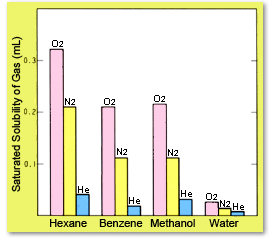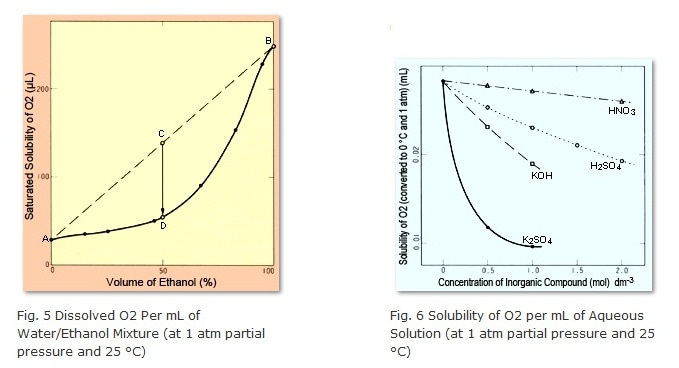# 2-3) Solvent Mixtures

Mechanism of How Air Bubbles Are Generated

The saturated solubility of gases varies depending on the type of liquid. In general, gases with lower polarity tend to dissolve more easily in solvents with lower polarity. Figure 4 shows that benzene and methanol have roughly the same dissolved gas per volume. If this is calculated in terms of the amount dissolved per mole solvent, in other words the mole fraction, it is clear that the lower the polarity of the solvent, the higher the dissolution rate.
What happens to the saturated solubility, then, if different solvents are mixed? The answer is that, in general, the amount dissolved in the mixed solution is less than the sum of the amounts for the individual solvents. Figure 5 shows the amount of dissolved O2 in a mixture of water and ethanol (at 1 atmosphere partial pressure). Point A indicates the amount of dissolved O2 in 100 % water, whereas Point B indicates the amount of dissolved O2 in 100 % ethanol.  When equal amounts of water and ethanol are mixed, the solution contains a quantity of O2 corresponding to Point C, but the saturated solubility for this solution is only at Point D. Therefore, the difference in O2 quantity between Points C and D is generated as bubbles.   This same thing happens for water/methanol and water/acetonitrile mixtures, with respect to the saturated solubility of air.

Note: 1 atmosphere (1 atm) = 1.013 × 105 PaFig. 4 Dissolved Gas per mL of Solvent (at 1 atm partial pressure and 25 °C)

Bubbles can even occur if both of the solvents being mixed are water. This is because the saturated solubility of air generally decreases with increasing of salt concentrations. However, that relationship is not linear, but rather curved downward, as shown in Figure 6.### Reference Documentation

S.R. Bakalyar, M.P.T Bradley, and R. Honganen, J. Chromatogr., 158, 277-293 (1978)
Kagaku Binran, Kiso-hen II 8.7 Yokaido (General Chemistry Handbook, Fundamentals vol. II, 8.7 Solubility), edited by the Chemical Society of Japan, published by Maruzen Co. Ltd. (1984)